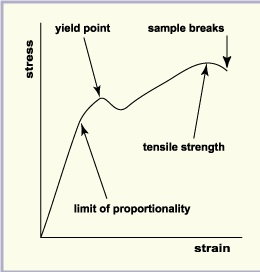Jump to content
Mechanical Engineering
• Sign Up

## Recommended Posts

Stress strain graph could be a behavior of fabric once it's subjected to load. during this diagram stresses area unit aforethought on the vertical axis and as a results of these stresses, corresponding strains area unit aforethought on the horizontal axis. As shown below within the stress strain curve.

#### Stress :

The term stress (s) is used to express the loading in terms of force applied to a certain cross-sectional area of an object. From the perspective of loading, stress is the applied force or system of forces that tends to deform a body.Strain Stress Graph
i.e. Stress = force / cross sectional area:
where,
σ = stress,
F = force applied, and
A= cross sectional area of the object.
Units of s : Nm-2 or Pa.

#### Strain:

Strain is the response of a system to an applied stress. When a material is loaded with a force, it produces a stress, which then causes a material to deform. Engineering strain is defined as the amount of deformation in the direction of the applied force divided by the initial length of the material.
Strain = extension / original length
where,
ε = strain,
lo = the original length
e = extension = (l-lo), and
l = stretched length

## Join the conversation

You can post now and register later. If you have an account, sign in now to post with your account.Reply to this topic...

×   Pasted as rich text.   Paste as plain text instead

Only 75 emoji are allowed.

×   Your link has been automatically embedded.   Display as a link instead

×   Your previous content has been restored.   Clear editor

×   You cannot paste images directly. Upload or insert images from URL.

×

×
×
• Create New...